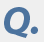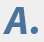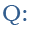Dear Dr. Don,
How does one convert APY to APR and vice versa? Are charts or formulas available?

Thanks,

Tony TimingDear Tony,
I keep the two straight by remembering that I pay a rate on my loans and earn a yield on my investments. So an annual percentage rate, or APR, is for loans and an annual percentage yield, or APY, is for investments.

Both rates have formulas based on legislation. The Truth in Lending Act, or TILA, spells out how an APR is calculated, while the Truth in Savings Act, or TISA, describes how an APY is calculated. The legislative approaches to calculating these rates were developed to make it easier for consumers to comparison shop loans or investments.

You can look to these
acts for a more in-depth description of calculating these percentages or download the calculators from the
Office of the Comptroller of the Currency, or OCC, but I’m going to assume that you’re not really concerned about the nuances of what expenses have to be included in these calculations and you just want to relate an APY to an APR.

One key difference between the two is that an APR doesn’t consider compounding. Ignoring the provisions in TILA and just considering interest expense, it takes the stated interest rate for a period and multiplies it by the number of periods in a year to arrive at the APR. If a credit card has a daily periodic rate of 0.05203 percent, for example, the APR is 0.05203% x 365 = 18.99 percent.

In contrast, the APY does consider compounding. It adds 1 to the periodic rate and raises that sum to the number of periods in a year. Then 1 is subtracted from the result to arrive at the APY. Using the credit card’s daily periodic rate of 0.05203 percent, the APY is: (1.0005203)
365 – 1 = 20.91 percent.

You can use Bankrate’s certificate of deposit
interest calculator to determine the APY on an investment. Since an APR is the periodic rate multiplied by the number of periods, no special calculator is needed to compute an APR.

So what’s key to your question of converting interest rates from APYs to APRs or vice versa is to be able to determine the periodic rate. That’s the interest rate used to calculate the interest earned/paid for a time period. It’s the nominal interest rate divided by the number of times interest is earned/paid in a year. A CD that pays interest monthly based on a 6 percent nominal interest rate pays a monthly (periodic) rate of 0.5 percent. The APR is 6 percent (0.5% x 12), but the APY is 6.17 percent ((1.005)
12-1).

To ask a question of Dr. Don, go to the ”
Ask the Experts” page, and select one of these topics: “financing a home,” “saving & investing” or “money.”

## You may also like

•## Banks offer plenty of CD options to suit your needs

•## Difficult to predict how rates will move

•## Sinking CD yields may not pay mortgage

•## The best financial moves to make now

•## Deposit safe if FDIC insured

•